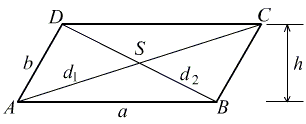# Every Parallelogram Is a Rectangle

Given parallelogram ABCD we denote two of its opposite sides a and the other b. The diagonals are d1 and d2.Each of the diagonals of a parallelogram divide it into two equal triangles, implying that the areas of the four triangles ABC, BCD, CDA, and DAB, are all equal.

Let denote H(p, q, r) Heron's formula for a triangle with sides p, q, r.

Consider triangles ABC and DAB; there sides are a, b, d1 and a, b, d2, respectively. The equality of the areas could be expressed in terms of Heron's formula:

H(a, b, d1) = H(a, b, d2),

from which we conclude that d1 = d2. However, a parallelogram with equal diagonals is necessarily a rectangle.

This conundrum is due to Vladimir Nikolin, an elementary school teacher from Serbia.•